# Precalculus : Evaluate a Polynomial Using Synthetic Substitution

## Example Questions

### Example Question #461 : Pre Calculus

Using synthetic division determine which of these is a factor of the polynomial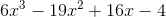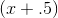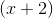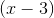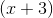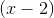Explanation:

Synthetic division is a short cut for doing long division of polynomials and it can only be used when divifing by divisors of the form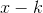. The result or quoitient of such a division will either divide evenly or have a remainder. If there is no remainder, then the "" is said to be a factor of the polynomial. The polynomial must be in standard form (descending degree) and if a degree is skipped such as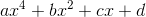it must be accounted for by a "place holder".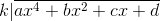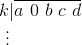___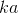__ __ ____ __ ___whereis the remainder.

While doing the long division we add vertically and we multiply diagonally by k. The empty lines represent places we put the sums and products. Notice that after the first term in the top row there is a 0; this is the place holder. This is because the degrees in the polynomial skipped. When the new coefficients have been found always rewrite starting with one order lower than the highest degree of the original polynomial.

Use synthetic division to verify each factor of the form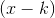. Lets start with.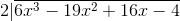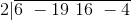Two goes into 6 three times resulting in: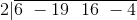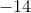_____________________From here we seewill give you a remainder of zero and is therefore a factor of the polynomial.

### Example Question #462 : Pre Calculus

Which of the following is the correct answer (quotient and remainder format) for the polynomial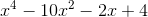being divided by.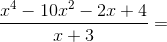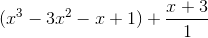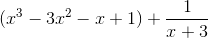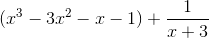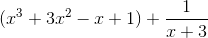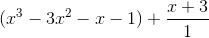Explanation:

Recall that dividing a polynomial bydoes not always result in a pefect division (remainder of 0). Sometimes there is a remainder just like in normal division. When there is a remainder, we write the answer in a certain way.

For example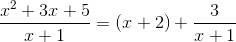where the divisor is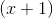, the quotient or answer is, the remainder is, and the dividend is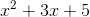.

Even though we have variables here, this is the same as noting that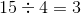with a remainder of.

And how do we check to know if we have the right answer? We multiply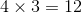and add 3 to get 15, our dividend. The same method is used for synthetic division.

Thus, for our problem:,

we must first multiply the divisor by the quotient using the foil method (first multiplying everything in the divisor by x and then everything in the divisor by 3)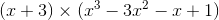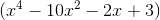now we just add the remainder which is 1 to yield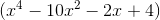which matches the original dividend and is therefore our answer!

### Example Question #21 : Polynomial Functions

Isa root of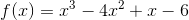?

Maybe

No

Yes

To determine ifis a root of the function given, you can use synthetic division to see if it goes in evenly. To set up the division problem, set up the coefficients of the function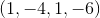and then set 1 outside. Bring down the 1 (of the coefficients. Then multiply that by thebeing divided in. Combine the result of that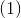with the next coefficient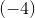, which is. Then, multiply that by. Combine that result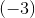with the next coefficient, which gives you. Multiply that by, which gives you. Combine that with the last coefficient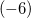, whcih gives you. Since this is not, you have a remainder, which means thatdoes not go in evenly to this function and is not a root.